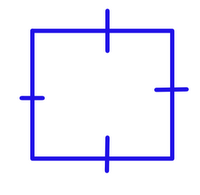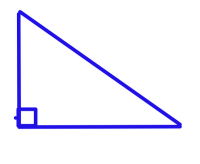### Pythagoras

Monday, February 23, 2009The statue is greek man, Pythagoras. He invented the pathagorean theorem. He like to play with music, make big words, and math. He dies at the age 90 or 100. he is related to everything, because he made the things that go together with each other.The pathagorean theorem was made by pathagaros. This is used to find out the side of a right angle triangle. You need to know 2 side lengths of the triangle to do it. By putting the length of a side of the triangle in the right letters spot, you can find out the side you don't know. It uses the idea of making a side into a square, then finding the square root. It's related to pythagoras becaus ehe made it, it is used for triangles, and you can make two triangles go together and make a square.This is a square. It is not a rectangle because it has lines of symetry. That means the sides are the same size. It has 4 right angles and is made out of 2 right angle triangles. Each corner is 90 degrees, thewhole thing is 360 degrees. It's related to the right angle triangle because it is made of 2, pythagorean theorem because you can do it to find out thr triangle and the square has 2 triangles, pythagaros because he mad e the theorem.This is a R.A.T. That means Right Angle Triangle. It is made of one 90 degree angle. There are side a and b . They are the base and straight line going up and down. Side c is the long one going crooked. The hypotenuse is accross the right angle sign. Theta and Beta make the 90 degree angle. It's related to the square because 2 of them make a square, pythagaros because he made the theorem for it, the theorem because its used for right angle triangles.